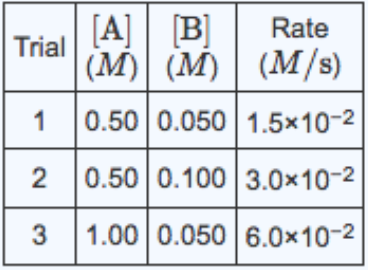# Problem: Consider the reaction A + 2B ⇌ C whose rate at 25°C was measured using three different sets of initial concentrations as listed in the following table: a) What is the rate law for this reaction? Express the rate law symbolically in terms of k, [A], and [B]. b) Calculate the initial rate for the formation of C at 25°C, if [A] = 0.50 M and [B] = 0.075 M. Express your answer to two significant figures and include the appropriate units.

###### FREE Expert Solution
100% (166 ratings)
###### FREE Expert Solution
100% (166 ratings)###### Problem Details

Consider the reaction A + 2B ⇌ C whose rate at 25°C was measured using three different sets of initial concentrations as listed in the following table:a) What is the rate law for this reaction? Express the rate law symbolically in terms of k, [A], and [B].

b) Calculate the initial rate for the formation of C at 25°C, if [A] = 0.50 M and [B] = 0.075 M. Express your answer to two significant figures and include the appropriate units.

What scientific concept do you need to know in order to solve this problem?

Our tutors have indicated that to solve this problem you will need to apply the Rate Law concept. You can view video lessons to learn Rate Law. Or if you need more Rate Law practice, you can also practice Rate Law practice problems.

What is the difficulty of this problem?

Our tutors rated the difficulty ofConsider the reaction A + 2B ⇌ C whose rate at 25°C was meas...as medium difficulty.

How long does this problem take to solve?

Our expert Chemistry tutor, Sabrina took 12 minutes and 1 second to solve this problem. You can follow their steps in the video explanation above.

What professor is this problem relevant for?

Based on our data, we think this problem is relevant for Professor Yamaguchi's class at Chaffey College.